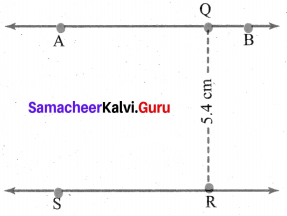## Tamilnadu Samacheer Kalvi 6th Maths Solutions Term 2 Chapter 4 Geometry Ex 4.2

Question 1.
Draw a line segment AB = 7 cm and Mark a point P on it. Draw a line perpendicular to the given line segment at P.
Solution:
Construction:
(i) Drawn a segment $$\overline{\mathrm{AB}}$$ such that $$\overline{\mathrm{AB}}$$ = 7 cm and took a point P anywhere on the line.
(ii) Placing the set square on the line in such a way that the vertex of its right angle coincides with P and one arm of the right angle coincides with the line AB.
(iii) Drawn a line PQ through P along the other arm of the right angle of the set square,
(iv) The line PQ is perpendicular to the line AB at P. ie PQ ⊥ AB and ∠APQ = ∠BPQ = 90°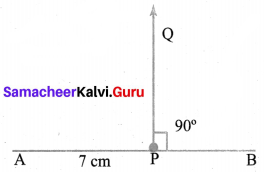Question 2.
Draw a line segment LM = 6.5 cm and take a point P not lying on it. Using set square construct a line perpendicular to LM through P.
Solution:
Construction:
(i) Drawn a line segment $$\overline{\mathbf{L M}}$$ such that $$\overline{\mathbf{L M}}$$ = 6.5 cm and marked a point P anywhere above $$\overline{\mathbf{L M}}$$
(ii) Placing one of the arms of the right angle of the set square along the line segment LM.
(iii) Sliding the set square along the line segment in such a way that the other arm of its right angle touches the point P. Draw a line along this side, passing through point P meeting $$\overline{\mathbf{L M}}$$ at Q.
(iv) The line PQ is perpendicular to the line segment LM. ie LM ⊥ PQ.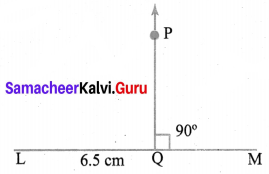Question 3.
Find the distance between the given lines using a set square at two different points on each of the pairs of lines and check whether they are parallel.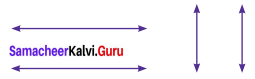Solution:
Making the points P, Q, R, S and A, B, C, D on the given lines
PQ = RS = 0.9 cm
AB = CD = 1 cm
Distance between the two lines are equal.
They are parallel lines.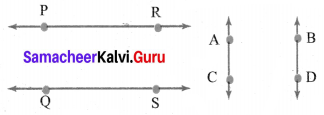Question 4.
Draw a line segment measuring 7,8cm. Mark a point B above it at a distance of 5 cm. Through B draw a line parallel to the given line segment.
Solution:
Construction:
(i) Using a scale drawn a line segment $$\overline{\mathrm{PQ}}$$ = 7.8 cm. Marked a point A on the line.
(ii) Placing the set square in such a way that the vertex of the right angle coincides with A and one of the edges of right angle lies along the line segment PQ. Mark a point B. Such that AB = 5 cm above the line PQ.
(iii) Placed the scale and the set square in such a way the set square is below PQ and one edge that form right angle with PQ. Placed the scale along the other edge of the right angle.
(iv) Holding the scale firmly and sliding the set square along the edge of the scale until the edge touches the point B. Drawn the line BC through B.
(v) Now the line BC is parallel to PQ i.e, BC || PQ.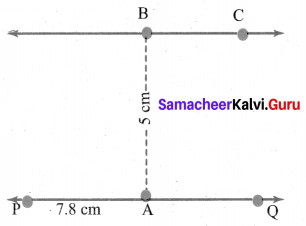Question 5.
Draw a line. Mark a point R below it at a distance of 5.4 cm. Through R draw a line parallel to the given line.
Solution:
(i) Using a scale drawn a line AB and marked a point Q on the line.
(ii) Placing the set square in such a way that the vertex of the right angle coincides with Q and one of the edges of the right angle lies along AB. Marked the Point R such that QR = 5.4 cm.
(iii) Placing the set square above AB in such a way that one of the edges that form a right angle with AB. Placed the scale along the other edge of the right angle.
(iv) Holding the scale firmly and slide the set square along the edge of the scale until the edge touches the point R. Drawn the line RS through R. The line RS is parallel to AB i.e RS || AB.